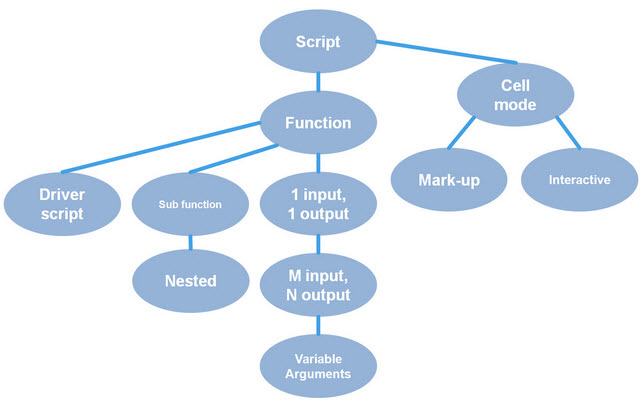Managing code in MATLAB: Functions of multiple inputs and outputs

This is the first series of MATLAB video tutorials that are an attempt to systematically make videos for a “technology trees” for using MATLAB. This first tree covers the various kinds of MATLAB files you might write. The tree shows you the order you should watch them because some rely on skills you need to learn from earlier videos, but some do not.This video will modify the function to accept more inputs and give more outputs.

|

コメント

コメントを残すには、ここ をクリックして MathWorks アカウントにサインインするか新しい MathWorks アカウントを作成します。